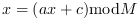Scilab Home page | Wiki | Bug tracker | Forge | Mailing list archives | ATOMS | File exchange
Change language to: Français - Português - 日本語 - Русский

See the recommended documentation of this function

# rand

Random numbers

### Syntax

```r = rand()
r = rand(m1,m2,...)
r = rand(m1,m2,...,key)
r = rand(x)
r = rand(x,key)

s = rand("seed")
rand("seed",s)

rand(key)
key = rand("info")```

### Arguments

m1, m2, ...

integers, the size of the random matrix `r`.

key

a string, the distribution of random numbers (default `key="uniform"`). The available values are `"uniform"` and `"normal"`.

x

a real or complex matrix. Only its real or complex content and its dimensions are taken into account.

r

a m1-by-m2-by-... real array of doubles with random entries.

s

a 1-by-1 matrix of doubles, integer value, positive, the seed (default `s=0`).

### Description

The purpose of this function is to return a matrix of doubles with real or complex random entries. Depending on the input arguments, the function can return a random matrix of doubles, or can get or set the distribution of random numbers, or can get or set the seed of the random number generator.

### Generate random numbers

r=rand()

returns a 1-by-1 matrix of doubles, with one random value.

r=rand(m1,m2)

is a random matrix with dimension `m1`-by- `m2`.

r=rand(m1,m2,...,mn)

returns a random matrix with dimension `m1`-by- `m2`-by-... -by-`mn`.

r=rand(a)

returns a random matrix with the same size as a. The matrix `r` is real if `a` is a real matrix and `r` is complex if `a` is a complex matrix.

### Change the distribution of the random numbers

The `key` input argument sets the distribution of the generated random numbers.

rand("uniform")

sets the generator to a uniform random number generator. Random numbers are uniformly distributed in the interval (0,1).

rand("normal")

sets the generator to a normal (Gauss-Laplace) random number generator, with mean 0 and variance 1.

key=rand("info")

return the current distribution of the random generator ("uniform" or "normal")

### Get or set the seed

It is possible to get or set the seed of the random number generator.

s=rand("seed")

returns the current value of the seed.

rand("seed",s)

sets the seed to `s` (by default `s=0` at first call).

### Examples

In the following example, we generate random doubles with various distributions.

```// Get one random double (based on the current distribution)
r=rand()
// Get one 4-by-6 matrix of doubles (based on the current distribution)
r=rand(4,6)
// Get one 4-by-6 matrix of doubles with uniform entries
r=rand(4,6,"uniform")
// Produce a matrix of random doubles with the same size as x
x=rand(4,4);
r=rand(x,"normal")
// Produce a 2-by-2-by-2 array of random doubles
r=rand(2,2,2)```

In the following example, we change the distribution of the number generated by `rand`. We first produce normal numbers, then numbers uniform in [0,1).

```// Set the rand generator to normal
rand("normal")
r=rand(4,6)
// Get the current distribution
key=rand("info")
// Set the rand generator to uniform
rand("uniform")
r=rand(4,6)
key=rand("info")```

In the following example, we generate a 2-by-3 complex matrix of doubles, with normal distribution.

```// Produce a 2-by-3 matrix of random complex doubles
x=rand(2,3)+%i*rand(2,3)
// Produce a matrix of random complex doubles with
// normal entries and the same size as x
r=rand(x,"normal")```

In the following example, we plot the distribution of uniform numbers produced by `rand`.

```r=rand(1000,1,"uniform");
scf();
histplot(10,r);
xtitle("Uniform numbers from rand","X","Frequency")```

In the following example, we plot the distribution of standard normal numbers produced by `rand`.

```r=rand(1000,1,"normal");
scf();
histplot(10,r);
xtitle("Normal numbers from rand","X","Frequency")```

### Get predictable or less predictable numbers

The "uniform" pseudo random number generator is a deterministic sequence which aims at reproducing a independent identically distributed numbers uniform in the interval (0,1).

In order to get reproducible simulations, the initial seed of the generator is zero, such that the sequence will remain the same from a session to the other. In other words, the first numbers produced by `rand()` always are : 0.2113249, 0.7560439, ...

In some situations, we may want to initialize the seed of the generator in order to produce less predictable sequences. In this case, we may initialize the seed with the output of the `getdate` function:

```n=getdate("s");
rand("seed",n);```

### The generator

The `"uniform"` random number generator is described in "Urand, A Universal Random Number Generator" by Michael A. Malcolm, Cleve B. Moler, Stan-Cs-73-334, January 1973, Computer Science Department, School Of Humanities And Sciences, Stanford University.

It is a linear congruential generator of the form :where the constants areAccording to the authors, this generator is a full length generator, that is to say, its period is.

The `"normal"` random number generator is based on the Box-Muller method, where source of the uniform random numbers is Urand.

### The statistical quality of the generator

Better random number generators are available from the grand function, in the sense that they have both a larger period and better statistical properties. In the case where the quality of the random numbers matters, we should consider the `grand` function instead. Moreover, the `grand` function has more features.

• grand — Random numbers
• ssrand — random system generator
• sprand — sparse random matrix